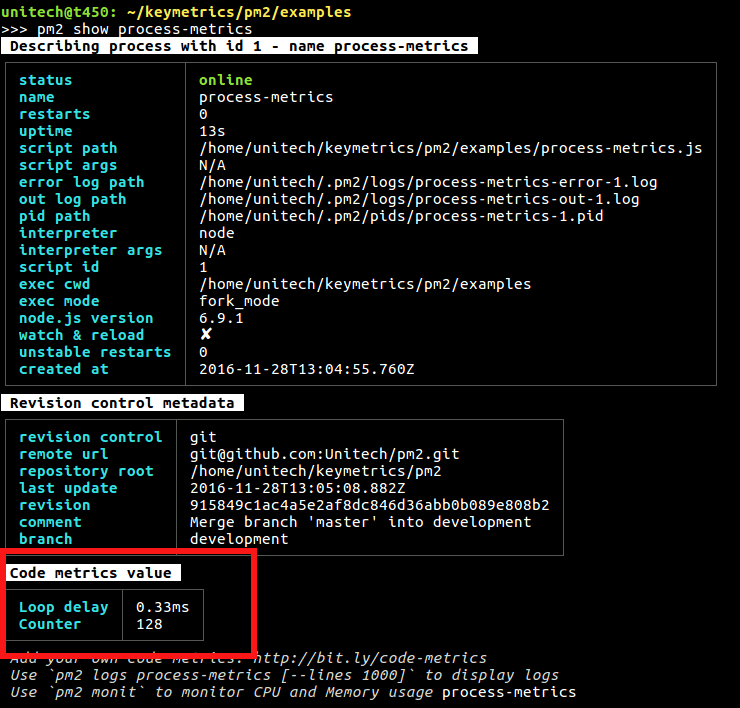# Custom Metrics

## Expose Metrics

By plugging custom metrics onto your code, you will be able to monitor in-code values, in realtime.

### Quick Start

First install tx2 module:

``````\$ npm install tx2
``````

Then create and app called monit.js:

``````const tx2 = require('tx2')
const http = require('http')

let meter = tx2.meter({
name      : 'req/sec',
samples   : 1,
timeframe : 60
})

http.createServer((req, res) => {
meter.mark()
res.write('Hello World!')
res.end()
}).listen(6001)
``````

And start it with PM2:

``````\$ pm2 start monit.js
``````

Now show the metrics with the command:

``````\$ pm2 show [app]
# pm2 show monit
``````

Note: metrics are in the section “Custom Metrics”.or you can use the Terminal based interface:

``````\$ pm2 monit
``````## Metrics helper available

Then you can program your very own metrics to track important information. 4 different probes are available:

• Simple metrics: Values that can be read instantly
• eg. Monitor variable value
• Counter: Things that increment or decrement
• Meter: Things that are measured as events / interval
• eg. Request per minute for a http server
• Histogram: Keeps a reservoir of statistically relevant values biased towards the last 5 minutes to explore their distribution
• eg. Monitor the mean of execution of a query into database

### API Documentation

Note: Refer to the TX2 API Documentation

### Examples

#### Simple Metric: Simple value reporting

This allows to expose values that can be read instantly.

``````const tx2 = require('tx2')

// Here the value function will be called each second to get the value
var metric = tx2.metric({
name    : 'Realtime user',
value   : function() {
return Object.keys(users).length
}
})

// Here we are going to call valvar.set() to set the new value
var valvar = tx2.metric({
name    : 'Realtime Value'
})

valvar.set(23)
``````

#### Counter: Sequential value change

Values that increment or decrement.

Example to count Active Http Requests:

``````const tx2 = require('tx2')
var http = require('http')

var counter = tx2.counter({
name : 'Active requests'
})

http.createServer(function (req, res) {
counter.inc()

req.on('end', function() {
// Decrement the counter, counter will eq 0
counter.dec()
})
res.write('Hello World!')
res.end()
}).listen(6001)
``````

#### Meter: Average calculated values

Values that are measured as events / interval.

Example to count number of queries per second:

``````const tx2 = require('tx2')
var http = require('http')

var meter = tx2.meter({
name      : 'req/sec',
samples   : 1,
timeframe : 60
})

http.createServer(function (req, res) {
meter.mark()
res.write('Hello World!')
res.end()
}).listen(6001)
``````
##### Options

samples option is the rate unit. Defaults to 1 sec. timeframe option is the timeframe over which events will be analyzed. Defaults to 60 sec.

#### Histogram

Keeps a reservoir of statistically relevant values biased towards the last 5 minutes to explore their distribution.

``````const tx2 = require('tx2')

var histogram = tx2.histogram({
name        : 'latency',
measurement : 'mean'
})

var latency = 0

setInterval(function() {
latency = Math.round(Math.random() * 100)
histogram.update(latency)
}, 100)
``````